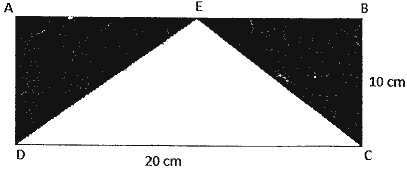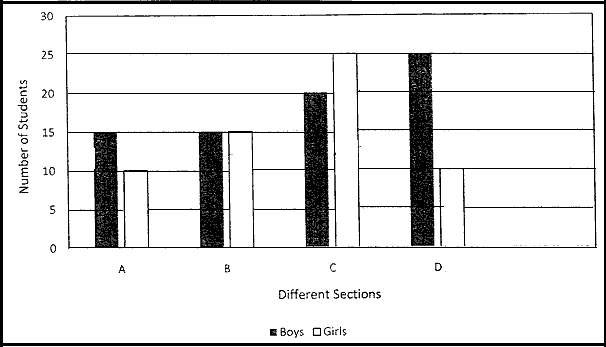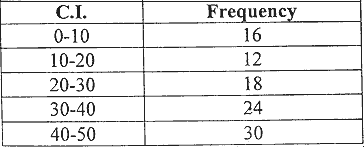Thursday , May 13 2021# 8th Mathematics Summative Assessment-II (2016-17)

## 8th Mathematics Summative Assessment-II (2016-17)

School Name: Venkateshwar Global School, Sector 13, Rohini, Delhi 110085 India
Time: 3 hour
M.M. 90 marks
Date: 28/02/2017
Subject: Mathematics
Class: VIII

## General Instructions:

• All questions are compulsory.

### 2 marks questions

#### Question: 7. Find the area of the shaded region if ABCD is a rectangle with length 20 cm and breadth 10 cm.#### Question: 10. Study the double bar graph and answer the questions that follow1. In which 2 sections are the number of boys equal?
2. How many sections have grater number of girls than boys?

### 3 marks questions

#### Question: 14.

1. Factorize: 4d2 – 8d + 3
2. Multiply: (4x2 – 7) by (2x + 3)

#### Question: 15. Find the mean of the following data#### Question: 16. A bag contains chits numbered from 20 to 79. Find the probability of getting

1. A chit numbered more than and equal to 75
2. A multiple of 10
3. A chit numbered less than 30

### 4 marks questions

1. 102 X 98
2.  (11)3

#### Question: 22. Simplify

1. (a + b – c)2 + (a + b)2
2. If x + y = 12 and xy = 14, find the value of (x2 + y2)

#### Question: 23. Factorize using appropriate identity

1. p3 – 25p
2. 25 x2 + 110 xy + 121 y2

#### Question: 28.

1. The ratio of 2 sides of a parallelogram is 4:3. If its perimeter is 56 cm, find the length of its.
2. Two adjacent angles of a parallelogram are (3x – 4) and (3x + 16). Find the value of x and hence find the measure of each of its angles.

#### Question: 31. A well shuffled pack of 52 plying cards is taken. Find the probability of getting

1. either a King or a Queen
2. neither a Jack nor an Ace
3. a face card
4. a red colored ball

## 10th CBSE Mathematics Pre Board Exam 2020-21

10th CBSE Mathematics Pre Board Exam 2020-21 School Name: Himalaya Public School, Sector 13, Rohini, Delhi 110085 India Time: 3 …